# How to Sum Positive Numbers in Excel – Easy Formula

For someone fairly comfortable with Excel, summing up a list of cells is as easy as pie.

But when it comes to summing up cells based on a particular condition, you might need to add a little more knowledge of functions to your stack.

As hard as it may seem, filtering out and summing only positive numbers from a list of cells is actually quite easy.

No, you don’t need filters or complicated nested IFs for this. Excel is well equipped with formulas to let you quickly sum positive numbers in a range while filtering out all the negatives.

In this tutorial we will show you two ways in which you can sum only positive numbers in Excel:

It’s time to let negativity go!

## Method 1: Calculate the Sum of Positive Numbers in Excel – Using SUMIF

Suppose you have a dataset as shown below and you want to sum all the positive numbers in column B.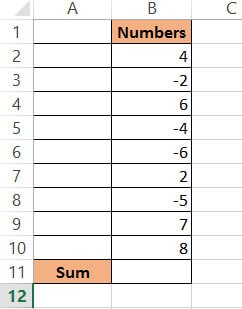Excel’s SUMIF function lets you add up numbers that match specified criteria in a range of cells. As such, this is the best way to selectively add up positive numbers.

Here’s the syntax for the SUMIF function:

`= SUMIF (range, condition,[sum_range])`

In this function,

• range is the range of cells containing the data you want the function to work on.
• condition is the condition that you want to be satisfied in order to include a cell in the sum.
• sum_range is an optional parameter. To avoid confusion, it is enough to know that you don’t really need it when finding the sum of positive numbers.

If all three parameters are given, then the SUMIF function checks each cell in the range to see if it matches the condition. If it does, then the function takes the corresponding cell value in sum_range and includes it in the sum.

If just the first two parameters are given, then the SUMIF function goes through each cell in the range and sums up only those cells that match the condition. It finally returns the sum of all cells in the given range that match the criteria.

If you want to find the sum of positive numbers, the condition should be “>0”, because, in order for a number to be considered as positive, it has to be more than 0.

So to sum up only positive values from the range B2:B10, the SUMIF function will be:

`= SUMIF(B2:B10, ">0")`

Notice that we did not include the third parameter here.

Let us see now how we can apply the SUMIF function, to sum up the positive numbers in our sample dataset:

1. Click on the cell where you want the result to be displayed. In our example, it will be cell B11.
2. Type the ‘equal to’ sign (=), followed by SUMIF and an opening bracket: =SUMIF(
3. Next, select the range of values that you want to find the sum from. In our example, you have to select cells B2 to B10.
4. A reference to cells B2: B10 should appear after the opening bracket in cell B11.
5. Insert a comma (,) followed by the condition “>0”
6. Insert a closing bracket. Your complete formula in cell B11 for our example should be =SUMIF(B2:B10, “>0”).
7. Press the Return key.

You should now see the resulting sum of positive numbers in cell B11.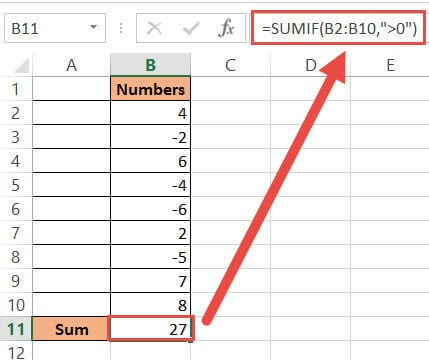In case you want to sum all the negative values, you can use the same formula with a minor change:

`= SUMIF(B2:B10, "<0")`

## Method 2: Adding only the Positive Numbers using VBA

If you are more of a coder at heart, then this method is for you.

Using VBA, you can create a macro to quickly sum up positive numbers in a range.

Here’s the VBA code that you can use:

```Sub Sum_only_positive_numbers()
Dim ws As Worksheet
Dim rng As Range
Dim result As Range
Set ws = Application.ActiveSheet
Set rng = Application.Selection
Set result = Application.InputBox( _
Title:="Get Location for Displaying Result", _
Prompt:="Select the cell where you want the result to appear", _
Type:=8)
result.Value = Application.WorksheetFunction.SumIf(rng, ">0")
End Sub```

This code takes a selection of cells and sums up the values of cells containing only positive numbers in that selection.

It then asks the user to select the cell where they want the result to appear. Once the user selects the cell, the code puts the result in the selected cell.

In case you need to sum all the negative numbers in a range in Excel, change the SumIf(rng, “>0”) part to SumIf(rng, “<0”) in the second-last line in the VBA macro code

1. From the Developer Menu Ribbon, select Visual Basic.
2. Once your VBA window opens, Click Insert->Module. Copy the code given above and paste it into the module window.
3. Your code is now ready to run and use whenever needed. Close the VBA window.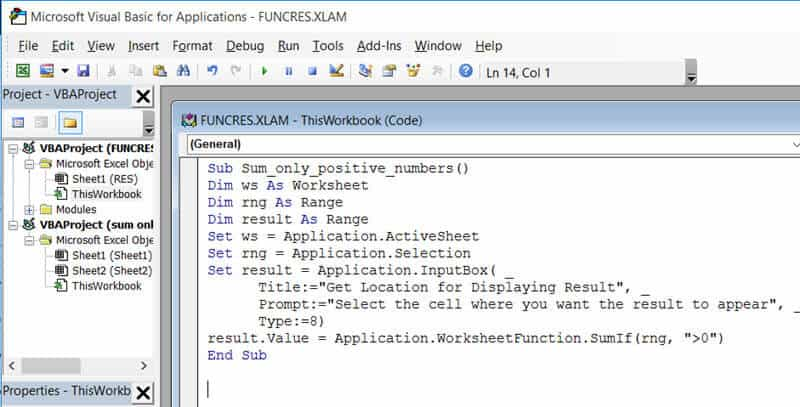4. Now go to your worksheet and select the range of cells containing the numeric values you want to work on (cells B2:B10 in our example).
5. Click on Macros, under the Developer tab.
6. This will open the Macros dialog box. Select the name Sum_only_positive_numbers from the list of macros displayed.
7. Click OK.
8. Your code should now run. You will see a prompt asking you to select the cell where you want the result displayed. Select cell B11 in our example.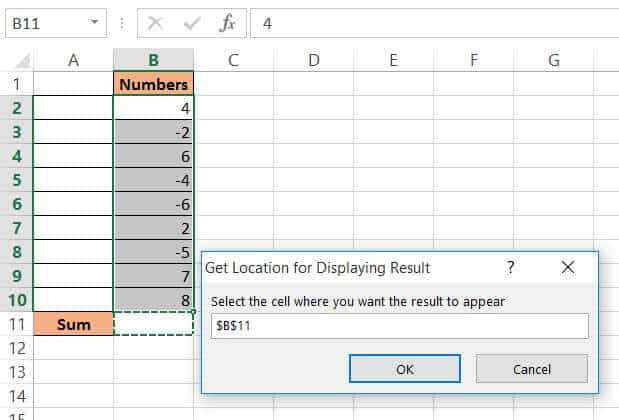9. You should see the result in your selected cell.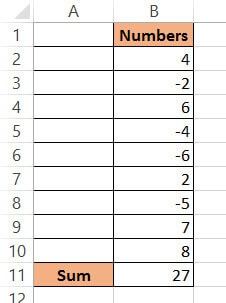Note that this method permanently changes the values in your worksheet. So there’s no way that you can undo it once the change is made.

Once you’re done writing the script you can re-use it as many times as you need to. For this, you can add the macro to your Personal Macro Workbook, attach it to a macro button or add it to your Quick Access Toolbar (QAT).

If you need to use this macro multiple times, you can create a quick access button. Using this button, you can quickly find the sum of positive numbers in a range of cells whenever you need to.

### Adding a Quick Access Button for the Macro

To add a button to quickly access the above macro, follow these steps:

1. Navigate to File->Options.
2. This will open the Excel Options dialog box. Select the Quick Access Toolbar option from the list on the left side of the dialog box.
3. Select Macros from the ‘Choose Commands From’ field.
4. Find the macro named Sum_only_positive_numbers from the list of macros on the left.
5. Click the Add button. This will add the macro Sum_only_positive_numbers to the Quick Access Toolbar. You should now see the macro’s name in the list on the right side of the dialog box.
6. Click OK to close the File Options dialog box.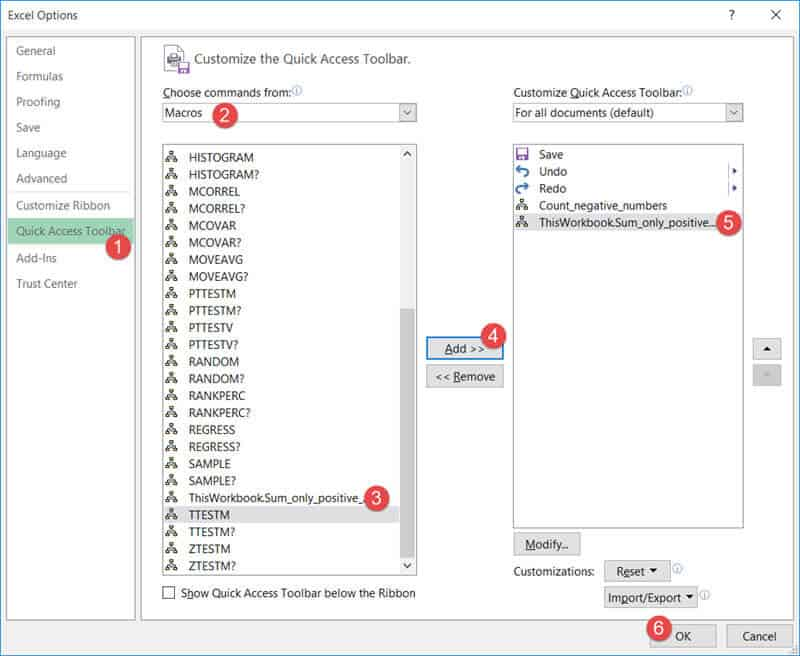7. You will now see that the macro icon has been added to the Quick Access Toolbar.Whenever you select a range of number cells and click on this quick access button, your code will run and you can use it to find the sum of positive numbers in your selected range.

In this tutorial, we saw two easy ways to selectively sum up the positive numbers in a range of cells. The first method involves the use of the SUMIF function with the condition “>0”. The second method involves the use of VBA code.

Note that you can select any range of numbers in any number of columns. The above two methods will help add up all the positive numbers in the entire selection.

Selectively summing up numbers need not always be complex. We hope this tutorial helped clear that out and gave you the confidence to try out more fun tricks with Excel.

Other Excel tutorials you may like: